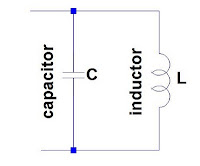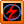### 0Online LC calculator 2

This is a simple LC calculator, for solving the value of inductance, capacitance  and frequency of an LC tuned circuit. This calculator is helpful specially in designing the value of  inductance in simple fm transmitters and radio related projects. It is similar to other LC calculator1 but in this version you can chose the units.

How to use:
1. Input two known values such as the frequency and inductance of the coil, or inductance of the coil and capacitance of capacitor,  and others.
2. Choose the units such as nano-farad for capacitance, MHz for frequency, and others.
3. Click calculate button to solve.
4.If you want to try again, click Clear button and input again.LC circuit

 Inductance nH pH µH mH H Capacitance pF nF µF mF F Frequency MHz Hz kHz GHz